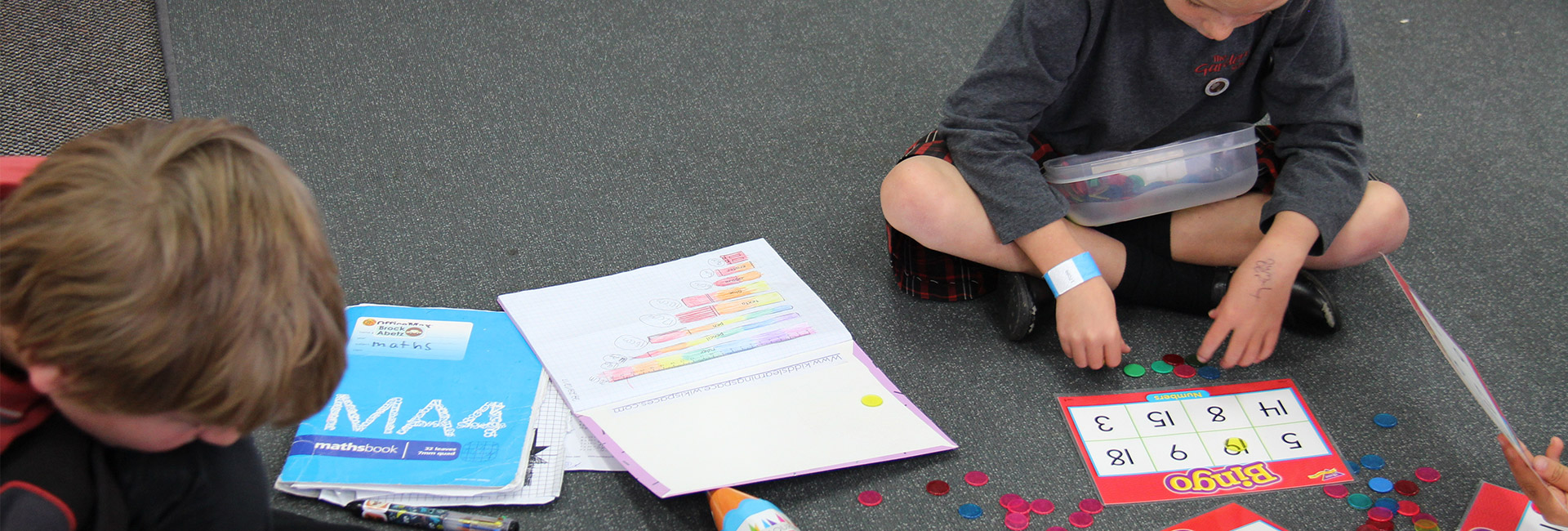# Year 3-4

## Maths Year 3 - 4

### What are the children learning?

After three years at school at school students will be applying basic addition facts and knowledge of place value and symmetry to: combine or partition whole numbers and find fractions of sets, shapes, and quantities.  By the end of Year 4 students be applying basic addition and subtraction facts, simple multiplication facts, and knowledge of place value and symmetry to: combine or partition whole numbers – find fractions of sets, shapes, and quantities.  In Years 3-4, Number is the focus of 60–80 percent of mathematics teaching time.

In Year 3-4 students may also be: comparing and sorting 3-dimensional shapes and objects.  Creating, comparing, continuing and finding the rule of sequential patterns. Using standard measurement tools, such as rulers and scales. As well as investigating statistical questions, gathering and displaying simple whole-number data.

### What does it look like in the classroom?

Students usually work in smaller maths groups based on their needs and abilities.  Mixed ability groups are introduced for some problem solving or strand maths tasks.   During the maths block students will work on a variety of mathematical tasks.  Each group has regular guided sessions with a teacher and opportunities to use their mathematical knowledge independently or in pairs and groups.  In addition they may have time on devices to complete Mathletics tasks, or time doing follow up book work tasks.

In Year 3 and 4 students are given an increasing number of opportunities to engage in mathematical problem solving and rich maths tasks, these require deeper thinking and looking for the most efficient way to solve everyday mathematical problems or challenges.